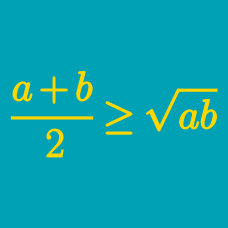Algebra

# Power Mean Inequalities Problem Solving

What is the minimum possible perimeter of a rectangle with area 25?

If $x^2 + y^2 = 1,$ find the maximum value of $(x + y)^2.$

Suppose that a company produces right-cylindrical soup cans with radius $r$ and $h$. If the volume is constrained to be a fixed value, which of the following dimensions minimizes the material per can?

Suppose $g(x)$ is positive for all $x$. Determine the minimum value of

$f(x) = \frac{16 (g(x))^2 + 1}{g(x)}.$

What is the minimum possible surface area of an open box (that is, a rectangular prism without its top) whose volume is $108$? (Count only the exterior surface area.)

×